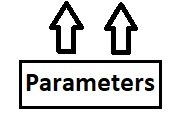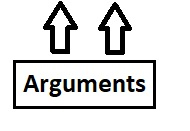# Functions in C/C++(3.5)

Functions are like a machine as they perform some functionality and produces a result of some type. Like, machine takes some input, process that input and produce an output similarly, function takes some value, operates on those value and produces the output. Manually a person passes the input to the machine then only the machine will start its functionality in the same manner when the programmer calls the function it will start executing.

Functions can be different in name in various languages, but they share two common characteristics like −

• They contain sequence of instructions that needs to be processed

• Those instructions are identified by a name, which refers to the function

## Why functions are used?

• Reusability− when the same functionality is required at multiple places the best approach will be creating the function once and call it multiple times instead of declaring the function providing same functionality again and again. The reusability is the biggest feature or advantage a function provides.

• Modularity to code − The function makes your coding neat and clean as instead of writing multiple lines of code in main() function, declare the functions to make it more clear and easy to read and code.

• Ease in modification − If there are any future changes to the code then instead of changing it to multiple places the programmer will make changes in the function only. Hence, we can say that function also reduces redundancy in the data.

• Provides Abstraction − With the relevant name of function it can determined what that function will supposed to do instead of revealing how that function is doing that. For example, in C we have “maths.h” header file that contains multiple function including pow() function. We can directly use this function to calculate the power value instead of knowing that how this function is calculating it in the definition.

## Function declaration and Definition

Function declaration is the process of telling the compiler the return type and the name of the function.

### Syntax

Without body

Return_type function_name(parameter list which is optional);

With body

Return_type function_name(parameter list which is optional)
{
//body of the function
} 

### Explanation

• return_type − It tells the compiler whether the function will return anything or not and if returns any data then what type of data it will return.

void dummy(){
//since the return type of function is void it willn’t return anything to the caller and hence it willn’t contain return statement.
}
Int dummy(){
//since the return type of function is int it will return integer value to the caller and it is mandatory that it will contain return statement as it is returning integer value.
return integer_value;
}
float dummy(){
//since the return type of function is float it will return floating value to the caller and it is mandatory that it will contain return statement as it is  returning floating value.
return float_value;
}

• function name − function name can be the any name programmer wants to give it to the function. Like in the above example, we have named the function as dummy

• parameters list(optional) − whenever the function will operate upon the passed values by the caller of the function, we need to create the parameters.

Function definition contains the functionality the function will supposed to do whenever it is called.

## Example

Live Demo

#include<iostream>
using namespace std;
//function that calculates the greatest
//value amongst two parameters
int greatest(int val_1, int val_2) //return type of function is integer value{
//body of the function(Definition)
if(val_1>val_2){
return val_1;
}
else{
return val_2;
}
}
int main(){
int val_1=10, val_2=20;
//calling the function and storing the integer value
//returned by a function in the integer variable
int highest = greatest(val_1,val_2);
//printing the greatest value
cout<<"The greatest value is : "<<highest;
//as the return type of main is int,
//it must have return statement for the compiler
return 0;
} 

## Output

The output for the above code will be −

The greatest value is : 20

## Function Parameters

• Parameters are optional, if there is no parameter then also function will perform its functionality

• Variables declared in the function definition to catch the values passed by the caller of the function is known as Parameters. int greatest(int val_1, int val_2)

int greatest(int val_1, int val_2)• Variables passed by the caller of the function are called as Arguments.

int highest = greatest(val_1,val_2);• Actual Parameters vs Formal Parameters-

Actual parameters are the data passed to the function like in above example, 10 and 20 are the actual parameters

Formal parameters are the data received by the function like in above example, val_1 and val_2 are the formal parameters.

## Important points about function main()

• Every program has a entry point from where the execution begins, like in C and C++ we have function main().

• If the main() function return type is void it means that function is not returning anything to the compiler whereas if the function main() have return type as int then it is returning value to the compiler. Like, we have “return 0” in main() which signifies the termination of a program.

• In C − The return type of function main() can be void and int only as main() function can either return integer value to the compiler or it shouldn’t return anything.

• In C++ − The return type of function main() can be int only as main() function returns integer value to the compiler.